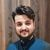# QlikView App Dev

Discussion Board for collaboration related to QlikView App Development.

Announcements
Join this live chat April 6, 10AM EST - QlikView to Qlik Sense REGISTER
cancel
Showing results for
Search instead for
Did you mean:Creator

## sum the value of distinct orders

Hi All,

Would really appreciate some help as I am stuck. I would like to sum a column named values for all distinct orders.

OrderReferenceValue
12
12
23
3

4

Basically the values for each order reference will be the same so I just want to sum the value for each unique order reference.

Would anyone be able to help me please? Also sum (distinct OrderRefernece) won't work as some order references will have the same value as another order reference.

Thanks,

Isaac

1 Solution

Accepted SolutionsMVP

May be this

Sum(Aggr(Value, OrderReference))

8 RepliesMVP

What is the expected output for the data you have provided above?Creator
Author

9 would be my ideal answer basically (2+3+4) ...any suggestions would be greatly appreciatedMVP

May be this

Sum(Aggr(Value, OrderReference))Creator
Author

Thanks Sunny, this has been really helpful. Can I just confirm my logic as to what's happening here?

Are we aggregating order reference and summing the value?

Thanks again for your help, really appreciate it.Creator II

In Sunny's expression we are aggregating 'Value on the field OrderReferenceMaster

Hi Isaac,

When you close the thread Sunny's answer should be marked as correct but in the meantime if I may I'd like to explain how the Aggr function is working here.

Aggr(Value, OrderReference) creates what may be described as an in-memory straight table with expression Only(Value) and dimension OrderReference. If you could see the table it would look like this:

OrderReference only(Value)
12
23
34

The Aggr function returns an array of values for Only(Value) - the values for each distinct OrderReference. You want the sum of these so we wrap the sum() function around the Aggr expression. It's a bit like setting the Total Mode in a straight table to the Sum of Rows:

OrderReference only(Value)
9
12
23
34

Just as you can set the Total Mode in a straight table to Avg, Min, Max, etc you can use the aggregation function of your choice to wrap around the Aggr expression.

Cheers

AndrewCreator
Author

Thank you Shashank Vyas, very helpfulCreator
Author

Thank you Andrew, really appreciate your explanation